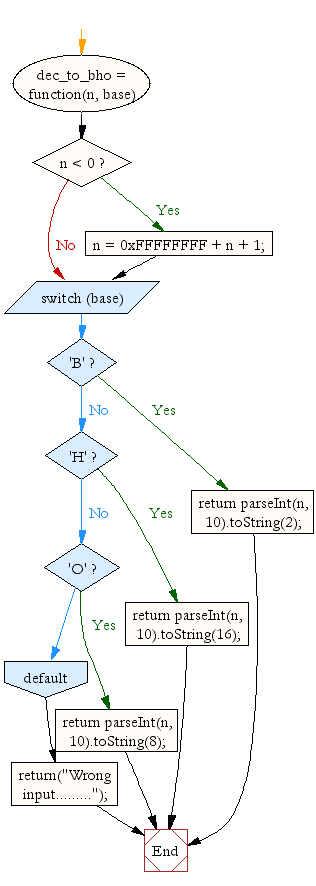# JavaScript: Convert a decimal number to binary, hexadecimal or octal number

## JavaScript Math: Exercise-3 with Solution

Write a JavaScript function to convert a decimal number to a binary, hexadecimal or octal number.

Test Data:
console.log(dec_to_bho(120,'B'));
console.log(dec_to_bho(120,'H'));
console.log(dec_to_bho(120,'O'));
"1111000"
"78"
"170"

Pictorial Presentation:Sample Solution:-

HTML Code:

``````<!DOCTYPE html>
<html>
<meta charset="utf-8">
<title>Convert a decimal number to binary, hexadecimal or octal number</title>
<body>

</body>
</html>
```
```

JavaScript Code:

``````dec_to_bho  = function(n, base) {

if (n < 0) {
n = 0xFFFFFFFF + n + 1;
}
switch (base)
{
case 'B':
return parseInt(n, 10).toString(2);
break;
case 'H':
return parseInt(n, 10).toString(16);
break;
case 'O':
return parseInt(n, 10).toString(8);
break;
default:
return("Wrong input.........");
}
}

console.log(dec_to_bho(120,'B'));
console.log(dec_to_bho(120,'H'));
console.log(dec_to_bho(120,'O'));
```
```

Sample Output:

```1111000
78
170
```

Flowchart:Live Demo:

See the Pen javascript-math-exercise-3 by w3resource (@w3resource) on CodePen.

Improve this sample solution and post your code through Disqus

What is the difficulty level of this exercise?

Test your Programming skills with w3resource's quiz.

﻿